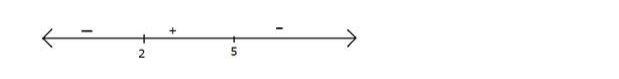# Solve

Question:

Solve $\frac{3}{x-2}<1$, when $x \in R$

Solution:

$\frac{3}{x-2}<1$

$\Rightarrow \frac{3}{x-2}-1<0$

$\Rightarrow \frac{3-x+2}{x-2}<0$

$\Rightarrow \frac{5-x}{x-2}<0$

Observe that $\frac{5-x}{x-2}$ is zero at $\mathrm{x}=5$ and not defined at $\mathrm{x}=2$

Hence plotting these two points on number line

Now for $x>5, \frac{5-x}{x-2}$ is negative

For every root and not defined value of $\frac{5-x}{x-2}$ the sign will changeWe want the negative part hence x < 2 and x > 5

x < 2 means x is from negative infinity to 2 and x > 5 means x is from 5 to infinity

Hence $x \in(-\infty, 2) \cup(5, \infty)$

Hence solution of $\frac{3}{x-2}<1$ is $x \in(-\infty, 2) \cup(5, \infty)$